# Operational calculus

Jump to: navigation, search

One of the methods of mathematical analysis which in many cases makes it possible to reduce the study of differential operators, pseudo-differential operators and certain types of integral operators (cf. Differential operator; Integral operator; Pseudo-differential operator) and the solution of equations containing them, to an examination of simpler algebraic problems. The development and systematic use of operational calculus began with the work of O. Heaviside (1892), who proposed formal rules for dealing with the differentiation operator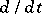and solved a number of applied problems. However, he did not give operational calculus a mathematical basis; this was done with the aid of the Laplace transform; J. Mikusiński (1953) put operational calculus into algebraic form, using the concept of a function ring. The most general concept of an operational calculus is obtained using generalized functions (cf. Generalized function).

The simplest variant of operational calculus is as follows. Letbe the set of functions (with real or complex values) given in the domainand absolutely integrable in any finite interval. The integral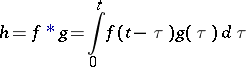is called the convolution of the functions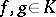. With the usual addition operation and the operation of convolution,becomes a ring without zero divisors (Titchmarsh's theorem, 1924). Elements of the quotient fieldof this ring are called operators and are written as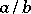; the fact that division inis not always possible is precisely the source of a new concept, operators, which generalizes the concept of a function. To indicate the necessary difference in operational calculus between the concepts of a function and of its value at a point, the following notation is used:## Contents

### Examples of operators.

1)is the integration operator: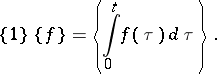Moreover,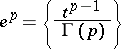and, in particular,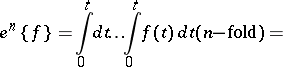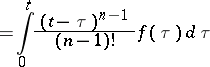This is the Cauchy formula, a generalization of which to the case of an arbitrary (non-integer) index serves to define fractional integration.

2)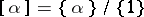(whereis a constant function) is a numerical operator; insofar as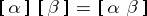,,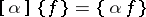, while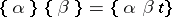, numerical operators behave as ordinary numbers. Thus the operator is a generalization not only of a function, but also of a number;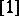is the unit of the ring.

3)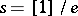is the differentiation operator, the inverse of the integration operator. So, if a functionhas a derivative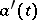, then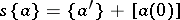and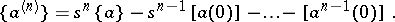Hence, for example,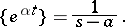Of course, a non-differentiable function can be multiplied by the differentiation operator; however, the result will be, in general, an operator.

4)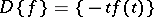is the algebraic derivative. It extends to arbitrary operators in the usual way. It appears that the action of this operator on a function of the differentiation operatorcoincides with differentiation with respect to.

Operational calculus provides suitable methods for the solution of linear differential equations, both ordinary and partial. For example, the solution of the equation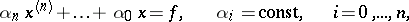satisfying the initial conditions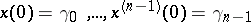automatically reduces to an algebraic equation. It is expressed symbolically by the formula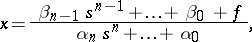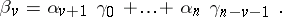The solution in its usual form is obtained by decomposition into elementary fractions with respect to the variable, with subsequent inverse transformation by referring to appropriate function tables.

In the use of operational calculus for partial differential equations (as well as for more general pseudo-differential equations), a differential and integral calculus of operator functions, i.e. functions with operator values, is employed. The concepts of continuity, derivative, convergence of series, integrals, etc., must be developed for these functions.

Let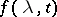be a function defined forand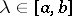. A parametric operator function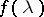is defined by the formula; it places operators of a certain type — functions in— in correspondence with the values ofbeing considered. An operator function is said to be continuous forif it can be represented as the product of an operatorand a parametric functionsuch that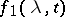is continuous in the ordinary sense.

### Examples.

1) Using the parametric function: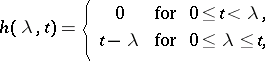the Heaviside function is defined: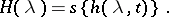The values of the hyperbolic exponential function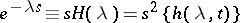are called shift operators, since multiplication of a given function by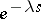requires the displacement of its graph overin the positive direction of the-axis.

2) The solution of the heat equation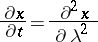can be expressed using the parabolic exponential function (which is also a parametric operator function):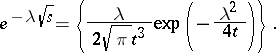3) A periodic function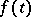with periodhas the representation:4) Ifhas numerical values in the interval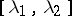, theni.e. multiplication of the given function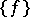bywith subsequent integration entails a truncation of its graph. In particular,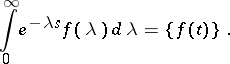Thus, with each functionfor which the integral being considered is convergent there is a corresponding analytic function: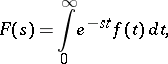its Laplace transform. As a result, a fairly broad class of operators is described by functions of one parameter; moreover, this formal similarity is defined more exactly in mathematical terms by establishing a definite isomorphism.

There are various generalizations of operational calculus; for example, operational calculus of differential operators other than, for example,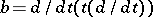, which is based on other function rings with a properly defined product.